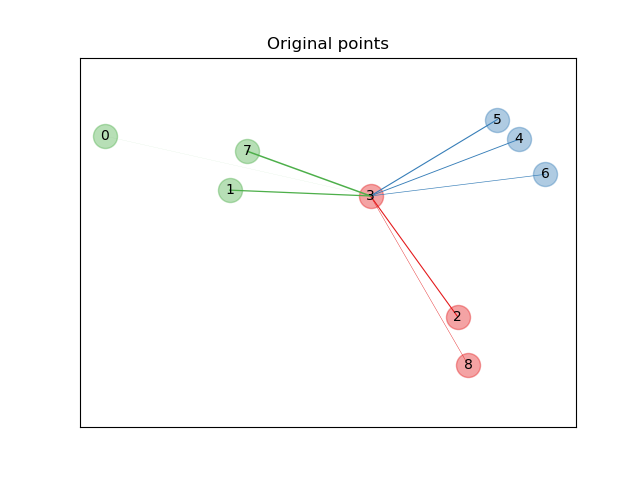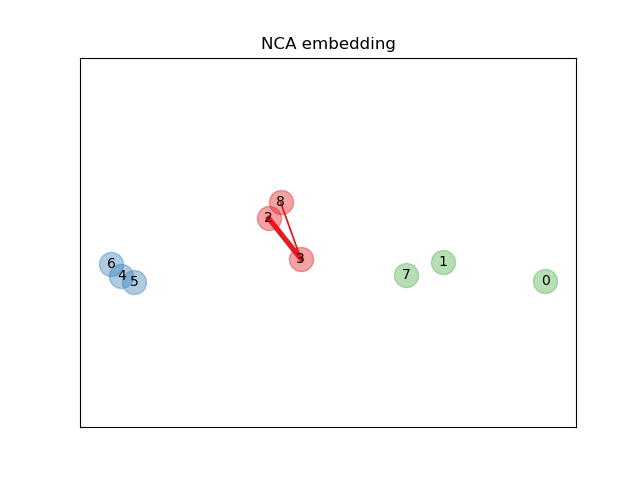# Neighborhood Components Analysis Illustration¶

This example illustrates a learned distance metric that maximizes the nearest neighbors classification accuracy. It provides a visual representation of this metric compared to the original point space. Please refer to the User Guide for more information.

# License: BSD 3 clause

import numpy as np
import matplotlib.pyplot as plt
from sklearn.datasets import make_classification
from sklearn.neighbors import NeighborhoodComponentsAnalysis
from matplotlib import cm
from sklearn.utils.fixes import logsumexp

print(__doc__)


## Original points¶

First we create a data set of 9 samples from 3 classes, and plot the points in the original space. For this example, we focus on the classification of point no. 3. The thickness of a link between point no. 3 and another point is proportional to their distance.

X, y = make_classification(n_samples=9, n_features=2, n_informative=2,
n_redundant=0, n_classes=3, n_clusters_per_class=1,
class_sep=1.0, random_state=0)

plt.figure(1)
ax = plt.gca()
for i in range(X.shape):
ax.text(X[i, 0], X[i, 1], str(i), va='center', ha='center')
ax.scatter(X[i, 0], X[i, 1], s=300, c=cm.Set1(y[[i]]), alpha=0.4)

ax.set_title("Original points")
ax.axes.get_xaxis().set_visible(False)
ax.axes.get_yaxis().set_visible(False)
ax.axis('equal')  # so that boundaries are displayed correctly as circles

diff_embedded = X[i] - X
dist_embedded = np.einsum('ij,ij->i', diff_embedded,
diff_embedded)
dist_embedded[i] = np.inf

# compute exponentiated distances (use the log-sum-exp trick to
# avoid numerical instabilities
exp_dist_embedded = np.exp(-dist_embedded -
logsumexp(-dist_embedded))
return exp_dist_embedded

def relate_point(X, i, ax):
pt_i = X[i]
for j, pt_j in enumerate(X):
if i != j:
line = ([pt_i, pt_j], [pt_i, pt_j])
ax.plot(*line, c=cm.Set1(y[j]),
linewidth=5*thickness[j])

i = 3
relate_point(X, i, ax)
plt.show()## Learning an embedding¶

We use NeighborhoodComponentsAnalysis to learn an embedding and plot the points after the transformation. We then take the embedding and find the nearest neighbors.

nca = NeighborhoodComponentsAnalysis(max_iter=30, random_state=0)
nca = nca.fit(X, y)

plt.figure(2)
ax2 = plt.gca()
X_embedded = nca.transform(X)
relate_point(X_embedded, i, ax2)

for i in range(len(X)):
ax2.text(X_embedded[i, 0], X_embedded[i, 1], str(i),
va='center', ha='center')
ax2.scatter(X_embedded[i, 0], X_embedded[i, 1], s=300, c=cm.Set1(y[[i]]),
alpha=0.4)

ax2.set_title("NCA embedding")
ax2.axes.get_xaxis().set_visible(False)
ax2.axes.get_yaxis().set_visible(False)
ax2.axis('equal')
plt.show()Total running time of the script: ( 0 minutes 1.758 seconds)

Estimated memory usage: 8 MB

Gallery generated by Sphinx-Gallery